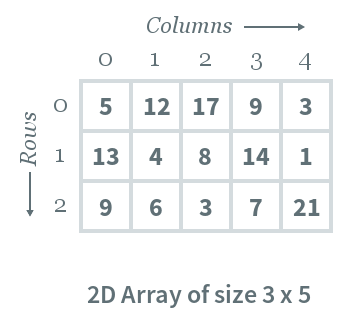Data Structures
Topics:
Multi-dimensional
• Arrays
• 1-D
• Multi-dimensional
• Stacks
• Queues
• Hash Tables
• Trees
• Disjoint Data Structures

# Multi-dimensional

A multi-dimensional array is an array of arrays. 2-dimensional arrays are the most commonly used. They are used to store data in a tabular manner.

Consider following 2D array, which is of the size $3 \times 5$. For an array of size $N \times M$, the rows and columns are numbered from $0$ to $N−1$ and columns are numbered from $0$ to $M−1$, respectively. Any element of the array can be accessed by $arr[i][j]$ where $0 \le i \lt N$ and $0 \le j \lt M$. For example, in the following array, the value stored at $arr[ 1 ][ 3 ]$ is $14$.2D array declaration:

To declare a 2D array, you must specify the following:
Row-size: Defines the number of rows
Column-size: Defines the number of columns
Type of array: Defines the type of elements to be stored in the array i.e. either a number, character, or other such datatype. A sample form of declaration is as follows:

type arr[row_size][column_size]

A sample C++ array is declared as follows:

int arr;


2D array initialization:
An array can either be initialized during or after declaration. The format of initializing an array during declaration is as follows:

type arr[row_size][column_size] = {{elements}, {elements} ... }


An example in C++ is given below:

int arr = {{5, 12, 17, 9, 3}, {13, 4, 8, 14, 1}, {9, 6, 3, 7, 21}};


Initializing an array after declaration can be done by assigning values to each cell of 2D array, as follows.

type arr[row_size][column_size]
arr[i][j] = 14


A C++ example of initializing an array after declaration by assigning values to each cell of a 2D array is as follows:

int arr;
arr = 5;
arr = 14;


This is quite naive and not usually used. Instead, the array elements are read from STDIN.

Processing 2D arrays:
The most basic form of processing is to loop over the array and print all its elements, which can be done as follows:

type arr[row_size][column_size] = {{elements}, {elements} ... }
for i from 0 to row_size
for j from 0 to column_size
print arr[i][j]


A C++ example of looping over the array and printing all its elements is as follows:

#include <iostream>
using namespace std;

int main()
{
// Array declaration and initialization
int arr = {{5, 12, 17, 9, 3}, {13, 4, 8, 14, 1}, {9, 6, 3, 7, 21}};
// Iterate over the array
for(int i=0; i<3; i++)
{
for(int j=0; j<5; j++)
{
// Print out each element
cout << arr[i][j];
}
// Print new line character after the row is printed in above loop
cout << endl;
}
return 0;
}

These methods of declaration, initialization, and processing can be extended to 3D or higher dimensional arrays.

Contributed by: Ravi Ojha
Уведомления

?